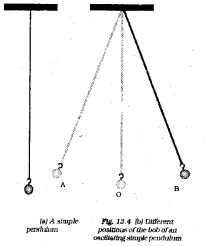# KSEEB Solutions for Class 7 Science Chapter 13 Motion and Time

Students can Download Chapter 12 Reproduction in Plants Questions and Answers, Notes Pdf, KSEEB Solutions for Class 7 Science, Karnataka State Board Solutions help you to revise complete Syllabus and score more marks in your examinations.

## Karnataka State Syllabus Class 7 Science Chapter 13 Motion and Time

### Class 7 Science Motion and Time NCERT Textbook Questions and Answers

Question 1.
Classify the following as motion along a straight line, circular or oscillatory motion:
(i) Motion of your hands while running.
Oscillatory motion.

(ii) Motion of a horse pulling a cart on a straight road.
Straight line motion.

(iii) Motion of a child in a merry-go-round.
Circular motion.

(iv) Motion of a child on a see-saw.
Oscillatory motion.

(v) Motion of the hammer of an electric bell.
Oscillatory motion.

(vi) The motion of a train on a straight bridge.
Straight-line motion.

Question 2.
Which of the following are not correct?
(i) The basic unit of time is second.
Correct.
(ii) Every object moves at a constant speed.
Not Correct.

(iii) Distances between the two cities are measured in kilometres.
Correct.

(iv) The time period of a given pendulum is not constant.
Not Correct.

(v) The speed of a train is expressed in m/h.
Not Correct.

Question 3.
A simple pendulum takes 32s to complete 20 oscillations. What is the time period of the pendulum?
Given
Number of Oscillations = 20
Total time taken to complete 20 oscillations = 32s.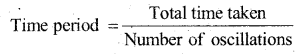Question 4.
The distance between two stations is 240 km. A train takes 4 hours to cover this distance. Calculate the speed of the train.
Given,
distance between the two stations = 240 km
Time taken = 4h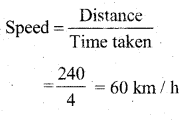Question 5.
The odometer of a car reads 57321.0 km when the clock shows the time at 08 : 30 AM. What is the distance moved by car, if at 08:50 AM, the odometer reading has changed to 57336.0 km? Calculate the speed of the car in km/min during this time. Express the speed in km/h also.
Given,
The initial reading of the odometer of the car = 57321.0 km.
The final reading of the odometer of the car = 57336.0 km
= 57336.0 – 5 7321.0 = 15 km
The given car starts at 8 : 30 a.m. and stops at 8 : 50 a.m.
Therefore, the time taken by the car to cover the distance is (8 : 50 – 8 : 30) min = 20 min.
i. e., distance = 15 km.
Time taken = 20 min
speed in km/min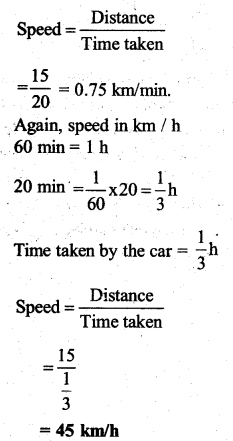Question 6.
Salina takes 15 minutes from her house to reach her school on a bicycle. If the bicycle has a speed of 2 m/s, calculate the distance between her house
Time taken by Salma to reach her school from her home = 15 min = 15 × 60 = 900s
Speed of her bicycle = 2m s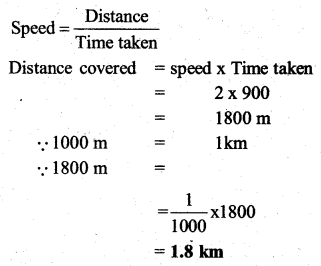Question 7.
Show the shape of the distance-time graph for die motion in the following cases:
(i) A car moving at a constant speed.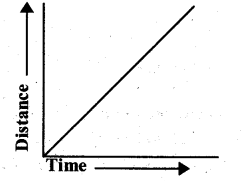(ii) A car parked on a side road.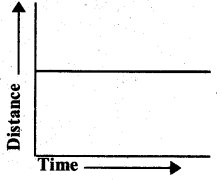Question 8.
Which of dying following relations is correct?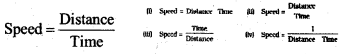Question 9.
The basic unit of speed is:
(i) km/min
(ii) m/min
(iii) km/h
(iv) m/s
(iv) m/s

Question 10.
A car moves with a speed of 40 km/h for 15 minutes and then with a speed of 60 km/h for the next 15 minutes. The total distance covered by the car is:
(i) 100 km
(ii) 25 km
(iii) 15 km
(iv) 10 km
Solution:
(ii) 25 km
Case I:
speed = 40 km / h
time = 15 min = 16/60 hour
Distance = speed × Time
$$=40 \mathrm{x} \frac{15}{60}$$
= 10 km,
speed = 60 km/h
Time = 15 min = 15/60 hour
distance = speed × Time
$$=60 \times \frac{15}{60}$$
= 15 km
Total distance = 10 km +15 km
= 25 km

Question 11.
Suppose the two photographs, shown in Fig. 13.1 and Fig. 13.2, had been taken at an interval of 10 seconds. If a distance of 100 meters is shown by 1 cm in these photographs, calculate the speed of the blue car.
From figures 13.1 and 13.2 we conclude that the distance covered by the blue car is 2 cm.
So, the distance covered = 2 × 100 m = 200m
Time Taken = 10 seconds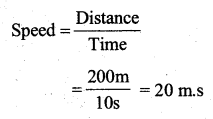Question 12.
Fig. 13.15 shows the distance-time graph for the motion of two vehicles A and B. Which one of them is moving faster?
Vehicle A is moving faster than the vehicle
Speed is given by the relation.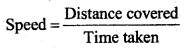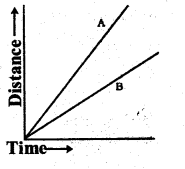This relation shows that the speed of a vehicle is greater if it covers a greater distances in a given interval of time. To compare the distance, draw a line perpendicular to the time – axis, as shown in the following distance-time graph.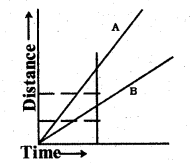From the graph, it is evident that for a given time t1, the distance covered by vehicle A is greater than that covered by vehicle B. Hence, vehicle A is moving faster than vehicle B.

Question 13.
Which of the following distance-time graphs shows a truck moving with a speed which is not constant?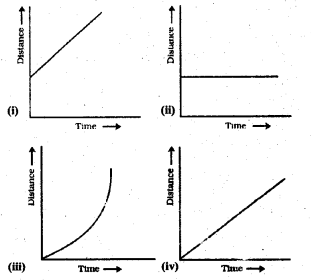### Class 7 Science Motion and Time Additional Important Questions and Answers

Question 1.
What is motion? How many types of motion? Name them.
The object or a person transfer from one place to another is known as motion. There are 3 types of motion.
They are

1. motion along a straight line
2. Circular and
3. periodic

Question 2.
Define speed, what is the unit of speed?
The distance covered by an object in unit time is known as speed.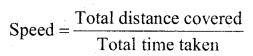The unit of speed is meter/second.

Question 3.
How do you measure the time?
Time is measured by using the oscillatory motions of the pendulum. The clocks and watches are measures the time.

Question 4.
Define the time period?
The time taken by the pendulum to complete one oscillation is called its time period

Question 5.
How do you write the symbols?
The symbols of all units are written in the singular.
For eg: 60 km not 40 kms.

Question 6.
Name the devices used before the pendulum clocks.
Sundial, water clocks, Sand clocks are some examples of devices used to measure the time in olden times.
Different designs of these devices were developed in different parts of the world.

Question 7.
What are the uses of the distance-time graph?
The uses of distance-time graphs are :

1. The nature of motion is given at a glance.
2. The relative motion at various intervals can
3. The region of acceleration or retardation can be estimated without any calculation.
4. The distance of the moving body at any time unit can be calculated.

Question 8.
Define speedometer and odometer.
A speedometer is a device in vehicles it records the speed of the vehicle directly in kilometer/hour. An odometer that measures the distance travelled (moved) by the vehicle.

Question 9.
Define uniform and non – uniform motion.